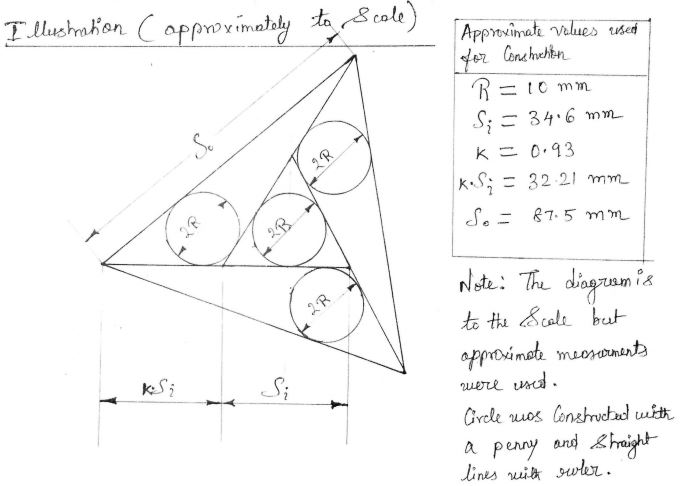# Four Incircles in an Equilateral Triangle, Third Solution

Elsewhere we described and solved the following sangaku:

In an equilateral triangle $ABC$ the lines $BEF$, $CFD$, $ADE$ are drawn making equal angles with $AB$, $BC$, $CA$, respectively, forming the triangle $DEF$, and so that the radius of the incircle of triangle $DEF$ is equal to the radii of the incircles of triangles $ABE,$ $BCF,$ and $ACD.$Find $DE$ in terms of $AB$.

The solution below is by J. John Samuel (7 MAy, 2014). Like George Zettler, John first establishes the basic facts, viz.,

1. The area $A$ of a triangle with the semiperimeter $p$ and the inradius $r$ equals $A=pr.$
2. In an equilateral triangle the side length $a$ and the inradius $r$ are related through $a = 2\sqrt{3}r.$
3. With the reference to the above diagram, in $\Delta ABE,$ the altitude $h$ from vertex $B$ equals $\displaystyle h=\frac{\sqrt{3}}{2}BE.$

Next, with a change of notations reflected in the diagram below,John derives the expression

$\displaystyle \frac{S_{o}^{2}}{S_{i}^{2}}=3k(k+1)+1,$

where $S_{o}$ and $S_{i}$ are the side lengths of the $o$uter and the $i$nner equilateral triangles. The easiest way to see that is to observe that, say, in $\Delta ABE,$ the Law of Cosines gives

$S_{o}^{2}=((kS_{i})^{2}+(kS_{i})((k+1)S_{i})+((k+1)S_{i})^{2}=(S_{i})^{2}(k^{2}+k(k+1)+(k+1)^{2}).$

The final step is illustrated by a diagram and a moving observation:We may find the relationship between $S_{o}$ and $S_{i}$ in yet another way, through the area formula $A=pr:$

\displaystyle\begin{align} Area(\Delta ABE) &= \frac{1}{2}(S_{o}+kS_{i}+(k+1)S_{i})R\\ &=\frac{1}{2}\frac{S_{i}}{2\sqrt{3}}(S_{o}+(2k+1)S_{i})\\ &=\frac{S_{i}}{4\sqrt{3}}(S_{o}+(2k+1)S_{i}). \end{align}

On the other hand,

\displaystyle\begin{align} Area(\Delta ABE) &= \frac{1}{2}h\cdot AE\\ &=\frac{1}{2}\frac{\sqrt{3}}{2}(kS_{i})((k+1)S_{i})\\ &=\frac{\sqrt{3}}{4}S_{i}^{2}k(k+1). \end{align}

Comparing the two expressions, we obtain $S_{o}=S_{i}(3k^{2}+k-1),$ which leads to an equation for k:

$\displaystyle\frac{S_{o}^{2}}{S_{i}^{2}}=(3k^{2}+k-1)^{2}=3k(k+1)+1.$

This is a fourth degree equation. Undeterred, we proceed to simplify:

\displaystyle\begin{align} 3k(k+1)+1 &= (3k^{2}+k-1)^{2}\\ &=9k^{4}+6k^{3}-5k^{2}-2k+1, \end{align}

or, $9k^{4}+6k^{3}-8k^{2}-5k=0.$ $k=0,$ not fitting the context of the problem can be cancelled out: $9k^{3}+6k^{2}-8k-5=0.$ In addition, we may notice (and John does) that $-9+6+8-5=0,$ implying that $k=-1$ is another irrelevant root of the equation. Dividing by $k+1$ leaves $9k^{2}-3k-5=0,$ with the roots $\displaystyle\frac{3\pm\sqrt{189}}{18}=\frac{1\pm\sqrt{21}}{6}.$ Dropping the negative root, we are left with the only suitable solution

$\displaystyle k=\frac{1+\sqrt{21}}{6}.$

Now we are in a position to solve the problem:

$\displaystyle \frac{S_{o}}{S_{i}}=3k^{2}+k-1=\frac{3+\sqrt{21}}{3}.$

From this

$\displaystyle \frac{S_{i}}{S_{o}}=\frac{3}{3+\sqrt{21}}=\frac{3(\sqrt{21}-3)}{21-9}=\frac{\sqrt{21}-3}{4}.$

This agrees with the solutions of George Zettler and Angela Drei.